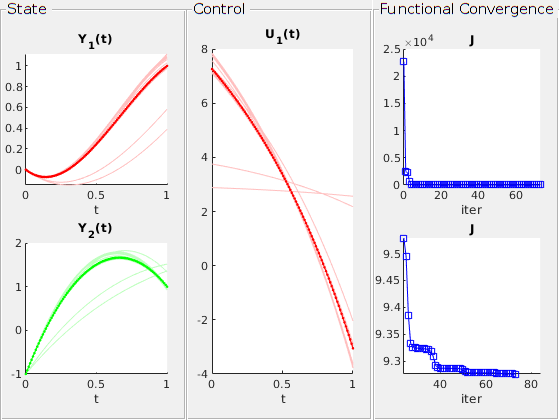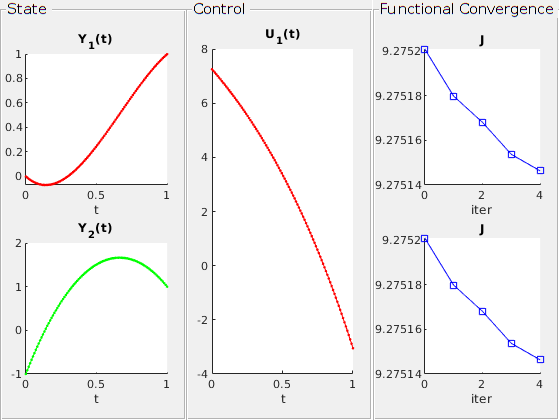# Indirect Methods

Gradient method is an iterative method finding optimal arguments. In the optimal control problem, it wants to find the optimal control vector $U$, in order to minimize the cost $J$.

Hence, in order to improve the value $J(U)$, gradient methods should estimate the directional derivative $dJ = J_U(U)$ and update the control vector along this direction based on a previous control vector $U_{old}$. There are different types of descent algorithms to update the control from the data $dJ$ and $U_{old}$.

DyCon toolbox has three implemented methods to find a proper descent.

• ‘ClassicalDescent’: After the gradient $dJ$ is calculated, it updates the control in the following way: $u_ {new} = u_ {old} + \ alpha dJ,$ where $\alpha$ is a constant.

• ‘AdaptativeDescent’: It updates the control in the same way as in ‘ClassicalDescent’. However, it first try the parameter $\alpha$ multiplied by two from the previous $\alpha$ and check whether the value of $J$ decreases. If not, it divide $\alpha$ into half and check whether if the value of J decreases. In this way, proper $\alpha$ will be determined to decrease $J$ effectively along the direction $dJ$.

• ‘ConjugateGradientDescent’: It updates the control as suggested in . It searches the direction of a linear combination between the gradient of the current control and the gradient of the previous control, in order to find a effective (conjugate) direction $J$ decreases. For the step-size $\alpha$, it calculate the minimal argument numerically using MATLAB built-in function.

The default methods of ‘Pontryagin’ class is ‘ConjugateGradientDescent’.

 : L. Lasdon, S. Mitter, and A. Waren. “The conjugate gradient method for optimal control problems.” IEEE Transactions on Automatic Control 12.2 (1967): 132-138.

In this tutorial, we present the use of different gradient methods in DyCon toolbox. We will implement various descent schemes on the following example problem: Minimizing the cost function

subject to

In order to construct ‘Pontryagin’ class, we define ‘ode’ class, the final cost ‘Psi’, and the running cost $L(t,Y,U)$ using symbolic variables and functions.

Y = sym('y',[2 1]); U = sym('u',[1 1]);

F = @(t,Y,U,Params) [ Y(2)           ; ...
-Y(2)      + U(1) ] ;

dynamics = ode(F,Y,U); %% Define 'ode' class
dynamics.InitialCondition = [0;-1];
dynamics.Nt = 100;

YT = [1;1];

Psi = @(T,Y) 5000*(Y-YT).'*(Y-YT);
L   = @(t,Y,U) 0.5*(U.'*U) + 0.5*(Y-YT).'*(Y-YT);

iP = Pontryagin(dynamics,Psi,L); %% Define 'OptimalControl' class
U0 = zeros(iP.Dynamics.Nt,iP.Dynamics.ControlDimension);


‘GradientMethod’ solves the optimal control problem of ‘Pontryagin’ class, where the default function is ‘@ConjugateGradientDescent’. In order to specify a descent algorithm, we use ‘DescentAlgorithm’ parameter of ‘GradientMethod’ function:

GradientMethod(iP,U0,'DescentAlgorithm',@ConjugateDescent) %% Same as default
plot(iP)

U1_tspan = iP.Solution.UOptimal;
Cost1 = iP.Solution.JOptimal;


Solve with precision:

We obtain: J(u) = 9.276146E+00

error = 3.189411E-01

With 74 iterations,     In 3.7135 seconds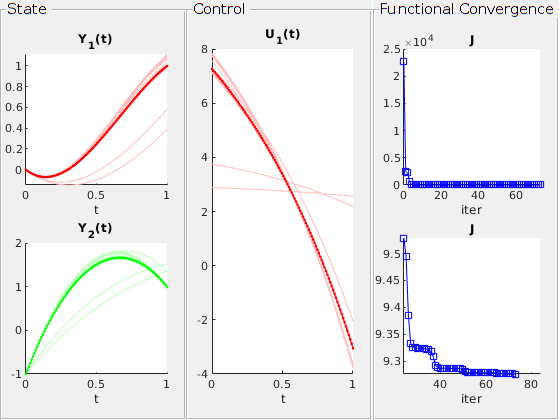GradientMethod(iP,U0,'DescentAlgorithm',@ClassicalDescent)
plot(iP)

Cost2 = iP.Solution.JOptimal;
U2_tspan = iP.Solution.UOptimal;


Solve with precision:

We obtain: J(u) = 9.275253E+00

error = 5.405759E-04

With 8 iterations,     In 0.19028 seconds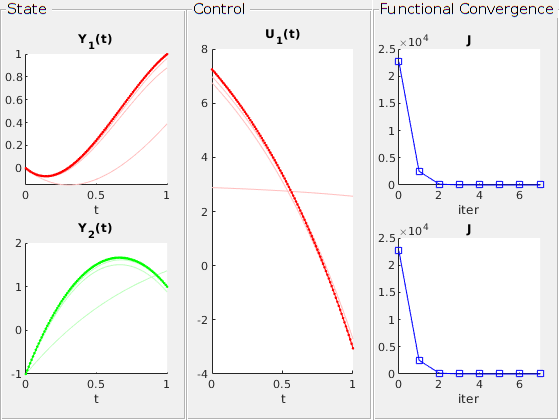GradientMethod(iP,U0,'DescentAlgorithm',@AdaptativeDescent)
plot(iP)
Cost3 = iP.Solution.JOptimal;
U3_tspan = iP.Solution.UOptimal;


Solve with precision:

We obtain: J(u) = 9.275201E+00

error = 1.813437E+00

With 53 iterations,     In 1.1484 seconds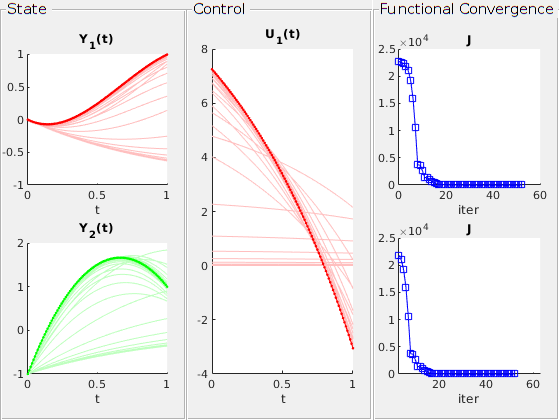clf;
tspan = iP.Dynamics.tspan;
plot(tspan,[U1_tspan],'r.--');
line(tspan,[U2_tspan],'Color','green','Marker','.');
line(tspan,[U3_tspan],'LineStyle','-','Color','blue','Marker','.');

xlabel('Time')
ylabel('Control')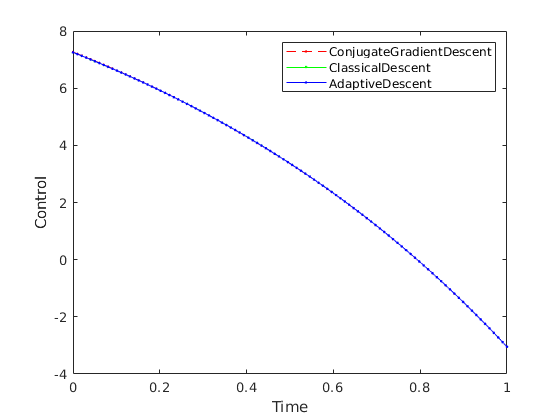We may use the options of ‘GradientMethod’, such as ‘Graphs’ or ‘U0’, where we may plot the figures during the calculation and provide initial guess on the control function by ‘U0’.

GradientMethod(iP,U3_tspan,'Graphs',true);


Solve with precision:

We obtain: J(u) = 9.275146E+00

error = 9.476464E-01

With 5 iterations,     In 0.48155 seconds